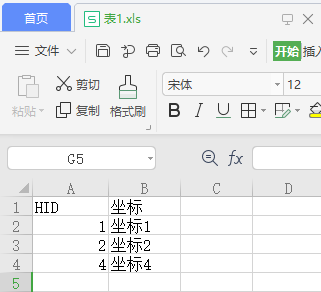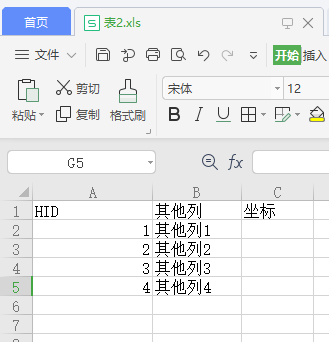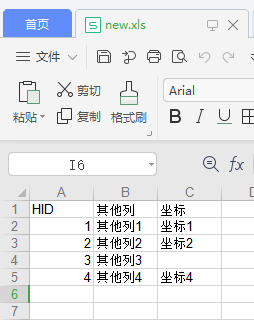• 主要介绍了利用Python实现Excel的文件间的数据匹配，本文通过一函数实现此功能，通过实例代码给大家介绍的非常详细，对大家的学习或工作具有一定的参考借鉴价值,需要的朋友可以参考下
• 需要根据HID匹配两个表，把表1的坐标内容补充到表2 代码 import shutil import sys import xlwt import xlrd file1 = "C:\\Users\\xuyin\\Desktop\\新建文件夹\\match-excel\\表1.xls" #打开表1 wb1 = xlrd.open...
需求背景
表1有两列表2包含表1不过缺少坐标字段需要根据HID匹配两个表，把表1的坐标内容补充到表2
代码
import shutil
import sys
import xlwt
import xlrd

file1 = "C:\\Users\\xuyin\\Desktop\\新建文件夹\\match-excel\\表1.xls"
#打开表1
wb1 = xlrd.open_workbook(filename=file1)
# 表1要匹配的列索引
hid_index1 = 0
# 表1目标数据列索引
target_index1 = 1
# 表1的sheet
sheet1 = wb1.sheet_by_index(0)
# 表1的sheet的总行数
rowNum1 = sheet1.nrows
# 表1的sheet的总列数
colNum1 = sheet1.ncols

file2 = "C:\\Users\\xuyin\\Desktop\\新建文件夹\\match-excel\\表2.xls"
#打开表2
wb2 = xlrd.open_workbook(filename=file2)#打开文件
# 表2要匹配的列索引
hid_index2 = 0
# 表2目标数据列索引
target_index2 = 2
# 表2的sheet
sheet2 = wb2.sheet_by_index(0)#通过索引获取表格
# 表2的sheet的总行数
rowNum2 = sheet2.nrows
# 表2的sheet的总列数
colNum2 = sheet2.ncols

# xlwt准备生成一个新的文件
write_workbook = xlwt.Workbook()

for index2 in range(0,rowNum2):
for col_index in range(0,colNum2):
# 遍历表2的每一行每一列,把对应的单元设置到新的文件中,即复制了表2的数据
write_sheet.write(index2,col_index,sheet2.cell_value(index2,col_index))
# 在遍历列过程中,如果碰到目标数据列索引.即需要补充的字段,则进行遍历表1,判断的id索引匹配
if col_index == target_index2:
for index1 in range(1,rowNum1):
hid1 = sheet1.cell_value(index1,hid_index1)
if hid1 == sheet2.cell_value(index2,hid_index2):
# 如果两个表的id相同则把表1的单元内容设置到表2对应的单元格
write_sheet.write(index2,col_index,sheet1.cell_value(index1,target_index1))

# 保存新的文件
write_workbook.save('new.xls')

结果展开全文• 两个表格，如图所示，按另一个表格某列的值对其中一个表格进行更新。 2.完整代码 可用完整代码如下 #!/usr/bin/python #coding=utf-8 ​ import xlrd #导入模块 import xlutils.copy ​ data_1 = xlrd.open_...
​1.题目
有两个表格，如图所示，按另一个表格某列的值对其中一个表格进行更新。2.完整代码
可用完整代码如下
#!/usr/bin/python
#coding=utf-8
​
import xlrd #导入模块
import xlutils.copy
​
data_1 = xlrd.open_workbook(r"F:\gang\0824\n.xls") #打开表
table_1 = data_1.sheets()
​
nrows_1 = table_1.nrows  #获取行数
print('行数为：',nrows_1)
​
data_2 = xlrd.open_workbook(r"F:\gang\0824\a.xls") #打开表
table_2 = data_2.sheets()
​
cols_2_f = table_2.col_values(1)  #获取某列值，以列表形式返回
print('FNODE_为：',cols_2_f)
cols_2_t = table_2.col_values(2)  #获取某列值，以列表形式返回
print('TNODE_为：',cols_2_t)
​
ws = xlutils.copy.copy(data_2) #对表2进行追加操作
table_3 = ws.get_sheet(0)
​
for i in range(1,nrows_1):
for j in cols_2_f[1:]:
if i == j:
table_3.write(j,8, table_1.cell_value(i,5))
table_3.write(j,10, table_1.cell_value(i,4))
print('第{}行结束'.format(i))
for k in cols_2_t[1:]:
if i == k:
table_3.write(k,11, table_1.cell_value(i, 5))
table_3.write(k,9, table_1.cell_value(i, 4))
print('第{}行结束'.format(i))
​
ws.save(r"F:\gang\0824\a.xls") #保存表
​
print('end job!!')

3.结果
执行结果和更新完的表格展示如下4.注意
①若缺少模块就安装模块
【ModuleNotFoundError: No module named ‘xlrd’】【ModuleNotFoundError: No module named ‘xlutils’】②把表格另存为【Excel97-2003工作簿（*.xls）】否则不能进行追加操作。
欢迎加群一起探讨细节python按文件名匹配文件，并复制到新文件夹中
python对文本文件的拆分展开全文• teacher = getnew(inpathnew) print(teacher) # print(nrows) for i in range(0, nrows): # 第0行为表头 if i == 10: break else: alldata = table.row_values(i) # 循环输出excel表中每一行，即所有数据 result = ...
import xlrd
def extract(inpath):
data = xlrd.open_workbook(inpath, encoding_override="utf-8")
table = data.sheets() # 选定表
nrows = table.nrows # 获取行号
ncols = table.ncols # 获取列号
all_info =[]
teacher = getnew(inpathnew)
print(teacher)
# print(nrows)
for i in range(0, nrows): # 第0行为表头
if i == 10:
break
else:
alldata = table.row_values(i) # 循环输出excel表中每一行，即所有数据
result = alldata + "、" + alldata + "、" + alldata + "、" + alldata + "、" + alldata # 取出表中第二列数据
name = "刘诗诗"
data = name in result
if data:
index = i
same_name = name
#print(result)
def getnew(inpathnew):
data = xlrd.open_workbook(inpathnew, encoding_override="utf-8")
table = data.sheets() # 选定表
nrows = table.nrows # 获取行号
ncols = table.ncols # 获取列号
all_info =[]
for i in range(1, nrows): # 第0行为表头
alldata = table.row_values(i) # 循环输出excel表中每一行，即所有数据
result = alldata
#result = alldata + "、" + alldata + "、" + alldata + "、" + alldata + "、" + alldata + "、" + alldata # 取出表中第二列数据
all_info.append(result)
print(all_info)
#all_info = (2, 5, 8, 10, 8, 12, 23, 3, 2, 3, 5)
import itertools
original_list = all_info
new_merged_list = list(itertools.chain(*original_list))
print(new_merged_list)
arr = new_merged_list
print(arr)
n = len(arr)
for i in range(0, n):
for j in range(i + 1, n):
if (arr[i] == arr[j]):
print("%s,%s" % (arr[i], arr[j]))
# # 定义列表
# l = ["a", "b", "c", "a", "b", "c", "a"]
# # 获取第一个"a"的下标
# index_one = l.index("a")
# # 从第一个"a"的下一个位置开始查找, 所以加1
# print(l.index("a", index_one + 1))
#return all_info
inpath = ("111.xlsx") # excel文件所在路径
inpathnew = ("222.xlsx") # excel文件所在路径
#extract(inpath)
getnew(inpathnew)
展开全文• excel处理大量数据匹配过程中，虽然可以使用vlookup，但是数据量超过10万进行批量匹配的时候，效率非常差，因此使用python。经查，发现python通过pandas库的merge可以实现类似于SQL中join的功能，具体参考下文： ...
在excel处理大量数据匹配过程中，虽然可以使用vlookup，但是数据量超过10万进行批量匹配的时候，效率非常差，因此使用python。经查，发现python通过pandas库的merge可以实现类似于SQL中join的功能，具体参考下文：

https://pandas.pydata.org/pandas-docs/stable/getting_started/comparison/comparison_with_sql.html#compare-with-sql-join

import pandas as pd
import numpy as np

# %%
with pd.ExcelFile('xx.xlsx') as xls:

outer=pd.merge(df1,df2,on='key')

outer.to_excel('outer_function.xlsx',index=False,encoding='utf-8')
最终实现Sheet1和Sheet2基于相同key字段的匹配，拼接。
不知道为啥，上面这个方法做出来总是有多有少，为了按顺序匹配，有遗漏的情况下我也好手动补充，采用了下面的方法，其中，结果的格式和内容顺序以df1为基础，可以方便的直接将遗漏的df1中的数据复制过来补充上。
outer=pd.merge(df1.drop_duplicates(),df2.drop_duplicates(),left_on='链接',right_on='链接',how='outer')

outer.to_excel(r'H:\e\outer_function3.xlsx',index=False,encoding='utf-8')

展开全文• # 循环输出excel表中每一行，即所有数据 result = alldata [ 0 ] + '、' + alldata [ 1 ] + '、' + alldata [ 2 ] + '、' + alldata [ 3 ] + '、' + alldata [ 4 ] # 取出表中第二...爬虫
• 今天小编就为大家分享一篇python进行两个表格对比的方法，具有很好的参考价值，希望对大家有所帮助。一起跟随小编过来看看吧
• 注：由于我们不是专门做数据处理与分析的，因此懂的也少，所以编写的python程序是简单易懂的...可以看到每个excel数据很多，都几千条，如果一个个看很费时。 import openpyxl # 加载第一个Excel data_raw= openpyxl..
• 需要处理一批EXCEL，需要将C列里面带有数据的，然后再去判断所在行的E列上一行减去下一行小于等于4的数据提取出来，重新写入一个EXCEL
• 工作中遇到要对两个Excel表格文件进行数据对比，找出其中不同的行。例如，比较同一用户的信息是否一致，这里的信息可能是不同版本的，但是属性列是一致的，数据内容上可能不同。如下表所示： Excel 1 姓名 性别...
• <code class="language-python">import xlrd def get_sensor_dict(filePath): excelfile = xlrd.open_workbook(filePath) sheets =...这执行出来只有a里面的数据，而提示b不支持匹配</p>有问必答
• 我们知道Excel有一match函数，可以做数据匹配。 比如要根据人名获取成绩 而参考表sheet1的内容如下： 要根据sheet1匹配每人的成绩，用Excel是这么写 index(Sheet1!B:B,MATCH(A2,Sheet1!A:A,0)) 意思就是获取...
• 打开excel表，需要在另一表中匹配相应学生姓名的学号信息。 之前尝试了excel中的VLOOKUP函数，试了很多次都没有成功，因此，用python试了一下 import pandas as pd subtrain = pd.read_csv('subtrainLabels.csv','...
• 有的时候，我们需要根据一个Excel中的某些信息，去另外一个Excel中查找某些信息，这该怎么实现呢？
• 相信有不少朋友日常工作会用到 Excel 处理各式表格文件，更有甚者可能要花大把时间来做繁琐耗时的表格整理工作。最近有朋友问可否编程来减轻表格整理工作量，今儿我们就通过实例来实现 Python 对表格的自动化整理。...
• 数据筛选： 根据实际需要输出筛选结果，或将筛选结果保存起来。总结起来可以分为三类： ① 将筛选的结果存入学过的数据结构里，比如：列表，元组或字典。 ② 将筛选的结果存入文件中。 ③ 将筛选的结果打印出来。 ...开发语言 后端
• 1.首先导入两个要用的包 import xlrd import openpyxl 2.测试表，也就是我们要对其进行写的表 data = openpyxl.load_workbook('测试.xlsx') sheetnames = data.get_sheet_names()#表名称列表 table = data.get_...列表
• 按某列ID合并两个Excel的数据，首先两个excel中要有...#按行匹配两个excel中的数据，以左边的表为基准 import pandas as pd datainfo1 = pd.read_excel(r'D:\Users\DUANPT\Desktop\datainfo1.xlsx') ort = pd.read_e
• 最近在工作中，需要人工比对大量的excel格式报表，刚好刚学了Pyhon入门基础知识，想着写东西练练手，不但能提高代码编写能力，还能减轻工作量，提高工作效率。说干就干，简单的理了逻辑。首先，将目标表和源表的...
• 两个文件，txt和excel 其中txt存放的是关键词，excel存放的是内容 txt存放关键词的形式是  成都 北京 天津 上海 重庆  excel存放的是一行一行的内容 能否将txt和excel进行匹配，提取...
• python 查找两个工作子表，两列的不同值 import xlrd #导入xlrd模块 data = xlrd.open_workbook(r'F:\desktop\工作簿.xlsm') #打开excel文件 data.sheet_names() print('sheet:'+str(data.sheet_names())) table...
• !... !... 我是一个自学Python的新手，现在想写大致如下的一个小程序。...我的思路是将两个EXCEL表格导入成两个字典，比较key，如果相同，则将value进行相乘，不知可不可行，有没有更好的方法，现在向专家们请教，感谢！
• 两个Excel中都有相同的一列，怎样依赖这列数据两个Excel合并到一起？使用Python合并表格只要一句代码！ 1 读取表格 使用Python读取数据只要3行，第一行载入pandas工具，第二行读取，第三行显示头部5行内容。 ...
• 日常中经常可能会遇到两个表格，或两个以上表格间的数据合并。如果两个表没有相互的联系，那直接合并即可。 但是，如果两个表格有一列是一样的，或者存在包含关系的，那这样随意的合并，就不那么的科学。 这篇文章...
• 核对Excel数据，获取查询列的对应值并填入。当在会议记录表A表中填入汇报人的名字时，能够根据名字在员工信息表B中查到这人所在的部门，并填到会议记录表A中。vscode
• 比如两Excel对应位置求和，并存储。（两Excel表格的第一列和第一行是一毛一样的，不需要求和，原样保存！） 本着能躺着就不坐着的原则，只想快点躺着，果断放弃了手工操作！！决心利用Python解决！！（可见会一门...
• 首先，将目标表和源表的内容分别写入到字典中，Excel表中不确定有没有字段是唯一值，所以选择了行号作为key值，一行的内容放到list中，然后从源表中取一行去目标表中遍历...#比对两个Excel文件内容的差异 #-------...
• 利用python处理excel数据：将其读取出来，并且最终实现数据相乘，打印出相乘的结果，其他的运算如加减都可以类比得出 实质在于，将其转化为一列表，接着进行赋值 以下的代码很简单，相当于一模板，在数据...
• 前言学习Python的过程中，我们会遇到Excel的各种问题。...Python解析Excel时需要安装两个包，分别是xlrd（读excel）和xlwt（写excel），安装方法如下：pip install xlrdpip install xlwt需求分析：......

# python匹配两个excel数据python 订阅